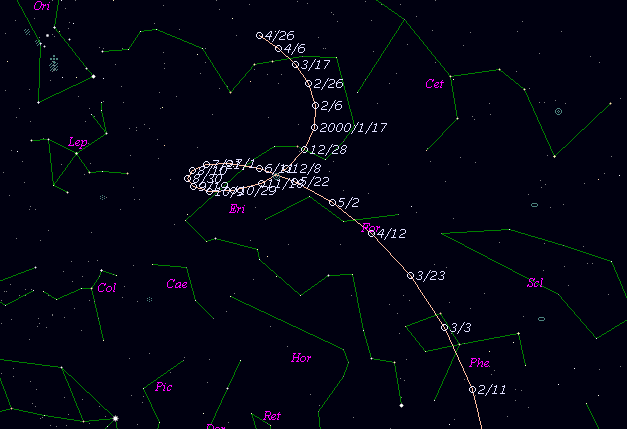# \$B%j%K%"WB@1(B

C/1998 K2 ( LINEAR )

 English version Home page Updated on January 26, 2000###\$B%W%m%U%#!<%k(B

 \$BH/8+F|(B 1998\$BG/(B5\$B7n(B22\$BF|(B \$BH/8+8wEY(B 15.7\$BEy(B \$BH/8+ Lincoln Laboratory Near-Earth Asteroid Research project

###\$B50F;MWAG(B

```   The following improved orbital elements by Kenji Muraoka, are from
180 observations  1998 May 22 to 1999 Dec. 3, including 9 Planets,
Moon and 5 minor planets perturbations and non-gravitational effect
of style II.   The mean residual is +/- 0.69 arc seconds.

Epoch  =  1998 Aug. 15.0  TT       JDT = 2451040.5
T  =  1998 Sept. 1.22545       +/- 0.00134 (m.e.) TT
Peri. =  221.41621                +/- 0.00063
Node  =   68.78093                +/- 0.00001   (2000.0)
Incl. =   64.45670                +/- 0.00005
q  =    2.3235003              +/- 0.0000066 AU
e  =    0.9993382              +/- 0.0000150
1/a  =   +0.0002848              +/- 0.0000065 1/AU
A1  =   -6.58                   +/- 2.29
A2  =  -12.3554                 +/- 1.8218
( P  = 208017 years )
```

###\$B@1?^(B###\$B8wEYJQ2=(B

```        m1 = 9.5 + 5 log\$B&\$(B + 5.0 log r
```##### \$B50F;MWAG\$OB<2,7r<#;a\$N7W;;\$K\$h\$k\$b\$N\$G\$9!#(B \$B@1?^\$O(B StellaNavigator Ver.2.0 for Windows (\$B%"%9%H%m%"!<%D(B \$BJTCx(B / \$B%"%9%-!<=PHG6I4)(B) \$B\$G:n@.\$7\$?\$b\$N\$G\$9!#(B \$B8wEY%0%i%U\$O(BComet for Windows\$B\$G:n@.\$7\$?\$b\$N\$G\$9!#(B